# NLP句子相似性方法总结及实现

1、基于Word2Vec的余弦相似度

2、TextRank算法中的句子相似性

3、莱文斯坦距离（编辑距离）

4、莱文斯坦比

5、汉明距离

6、Jaro距离（Jaro Distance）

7、Jaro-Winkler距离（Jaro-Winkler Distance）

8、基于Doc2Vec的句子相似度计算

### 1、基于Word2Vec的余弦相似度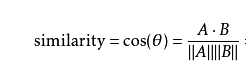#对每个句子的所有词向量取均值，来生成一个句子的vector
#sentence是输入的句子，size是词向量维度，w2v_model是训练好的词向量模型
def build_sentence_vector(sentence,size,w2v_model):
vec=np.zeros(size).reshape((1,size))
count=0
for word in sentence:
try:
vec+=w2v_model[word].reshape((1,size))
count+=1
except KeyError:
continue
if count!=0:
vec/=count
return vec

#计算两个句向量的余弦相似性值
def cosine_similarity(vec1, vec2):
a= np.array(vec1)
b= np.array(vec2)
cos1 = np.sum(a * b)
cos21 = np.sqrt(sum(a ** 2))
cos22 = np.sqrt(sum(b ** 2))
cosine_value = cos1 / float(cos21 * cos22)
return cosine_value

#输入两个句子，计算两个句子的余弦相似性
def compute_cosine_similarity(sents_1, sents_2):
size=300
vec1=build_sentence_vector(sents_1,size,w2v_model)
vec2=build_sentence_vector(sents_2,size,w2v_model)
similarity = cosine_similarity(vec1, vec2)
return similarity

### 2、TextRank算法中的句子相似性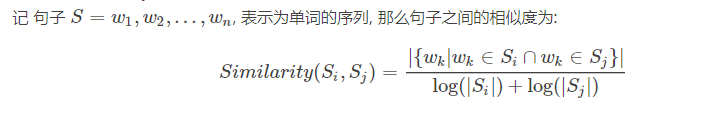def two_sentences_similarity(sents_1, sents_2):
counter = 0
for sent in sents_1:
if sent in sents_2:
counter += 1
sents_similarity=counter/(math.log(len(sents_1))+math.log(len(sents_2)))
return sents_similarity

### 3、莱文斯坦距离（编辑距离）

• 例如将kitten一字转成sitting：
• sitten（k替换为→s）
• sittin （e替换为→i）
• sitting （添加→g）
• 那么二者的编辑距离为3。

import Levenshtein
s1='kitten'
s2='sitting'
lev_distance=Levenshtein.distance(s1,s2)
print(lev_distance)

### 4、莱文斯坦比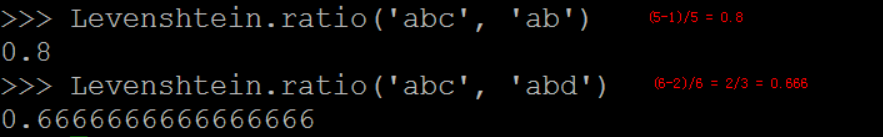### 5、汉明距离

import Levenshtein
s1='abc'
s2='cba'
lev_distance=Levenshtein.hamming(s1,s2)
print(lev_distance)
#结果输出为2

### 6、Jaro距离（Jaro Distance）

Jaro Distance 算法是一种计算两个字符串之间相似度的方法。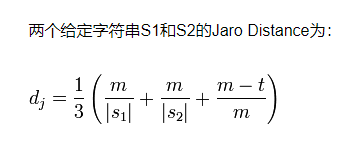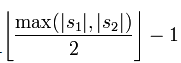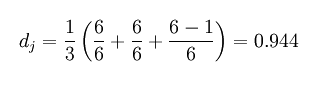import Levenshtein
s1='MARTHA'
s2='MARHTA'
lev_distance=Levenshtein.jaro(s1,s2)
print(lev_distance)
#结果输出为0.9444

### 7、Jaro-Winkler距离（Jaro-Winkler Distance）

Jaro-Winkler Distance给予了起始部分就相同的字符串更高的分数，他定义了一个前缀p。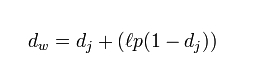import Levenshtein
s1='MARTHA'
s2='MARHTA'
lev_distance=Levenshtein.jaro_winkler(s1,s2)
print(lev_distance)
#结果输出为0.9611

### 8、基于Doc2Vec的句子相似度计算

from gensim.models.doc2vec import Doc2Vec

#推断一个句子的向量
sen_vec1=d2v_model.infer_vector('挺 菜品 单一 扇贝 好吃 水果 太少 服务 服务员 服务 挺 开心'.split())
print(sen_vec1)

#返回文档中和sen_vec句子最相似的前top个句子
sen_similar=d2v_model.docvecs.most_similar([sen_vec1],topn=3)
print(sen_similar)  #返回的是句子的编号和相似度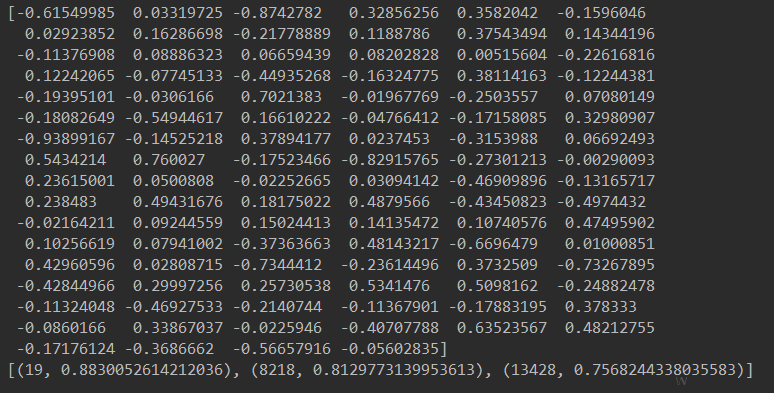1、Levenshtein全部函数链接

2、Jaro–Winkler distance链接

3、计算文本相似度工具fuzzywuzzy

​​​​​​​

01-16395412-021048
09-132951
04-251万+
03-071170
11-28377
07-119803
09-21
08-231625
06-041万+
07-051万+
12-031563
09-034956
05-134795
03-25
01-12